# Speaker Verification Using i-Vectors

Speaker verification, or authentication, is the task of confirming that the identity of a speaker is who they purport to be. Speaker verification has been an active research area for many years. An early performance breakthrough was to use a Gaussian mixture model and universal background model (GMM-UBM)  on acoustic features (usually `mfcc`). For an example, see Speaker Verification Using Gaussian Mixture Model. One of the main difficulties of GMM-UBM systems involves intersession variability. Joint factor analysis (JFA) was proposed to compensate for this variability by separately modeling inter-speaker variability and channel or session variability  . However,  discovered that channel factors in the JFA also contained information about the speakers, and proposed combining the channel and speaker spaces into a total variability space. Intersession variability was then compensated for by using backend procedures, such as linear discriminant analysis (LDA) and within-class covariance normalization (WCCN), followed by a scoring, such as the cosine similarity score.  proposed replacing the cosine similarity scoring with a probabilistic LDA (PLDA) model.  and  proposed a method to Gaussianize the i-vectors and therefore make Gaussian assumptions in the PLDA, referred to as G-PLDA or simplified PLDA. While i-vectors were originally proposed for speaker verification, they have been applied to many problems, like language recognition, speaker diarization, emotion recognition, age estimation, and anti-spoofing . Recently, deep learning techniques have been proposed to replace i-vectors with d-vectors or x-vectors  .

### Use an i-Vector System

Audio Toolbox provides `ivectorSystem` which encapsulates the ability to train an i-vector system, enroll speakers or other audio labels, evaluate the system for a decision threshold, and identify or verify speakers or other audio labels. See `ivectorSystem` for examples of using this feature and applying it to several applications.

### Develop an i-Vector System

In this example, you develop a standard i-vector system for speaker verification that uses an LDA-WCCN backend with either cosine similarity scoring or a G-PLDA scoring.Throughout the example, you will find live controls on tunable parameters. Changing the controls does not rerun the example. If you change a control, you must rerun the example.

### Data Set Management

This example uses the Pitch Tracking Database from Graz University of Technology (PTDB-TUG) . The data set consists of 20 English native speakers reading 2342 phonetically rich sentences from the TIMIT corpus. Download and extract the data set. Depending on your system, downloading and extracting the data set can take approximately 1.5 hours.

```url = 'https://www2.spsc.tugraz.at/databases/PTDB-TUG/SPEECH_DATA_ZIPPED.zip'; downloadFolder = tempdir; datasetFolder = fullfile(downloadFolder,'PTDB-TUG'); if ~exist(datasetFolder,'dir') disp('Downloading PTDB-TUG (3.9 G) ...') unzip(url,datasetFolder) end```
```Downloading PTDB-TUG (3.9 G) ... ```

Create an `audioDatastore` object that points to the data set. The data set was originally intended for use in pitch-tracking training and evaluation, and includes laryngograph readings and baseline pitch decisions. Use only the original audio recordings.

```ads = audioDatastore([fullfile(datasetFolder,"SPEECH DATA","FEMALE","MIC"),fullfile(datasetFolder,"SPEECH DATA","MALE","MIC")], ... 'IncludeSubfolders',true, ... 'FileExtensions','.wav'); fileNames = ads.Files;```

The file names contain the speaker IDs. Decode the file names to set the labels on the `audioDatastore` object.

```speakerIDs = extractBetween(fileNames,'mic_','_'); ads.Labels = categorical(speakerIDs); countEachLabel(ads)```
```ans=20×2 table Label Count _____ _____ F01 236 F02 236 F03 236 F04 236 F05 236 F06 236 F07 236 F08 234 F09 236 F10 236 M01 236 M02 236 M03 236 M04 236 M05 236 M06 236 ⋮ ```

Separate the `audioDatastore` object into training, evaluation, and test sets. The training set contains 16 speakers. The evaluation set contains four speakers and is further divided into an enrollment set and a set to evaluate the detection error tradeoff of the trained i-vector system, and a test set.

```developmentLabels = categorical(["M01","M02","M03","M04","M06","M07","M08","M09","F01","F02","F03","F04","F06","F07","F08","F09"]); evaluationLabels = categorical(["M05","M010","F05","F010"]); adsTrain = subset(ads,ismember(ads.Labels,developmentLabels)); adsEvaluate = subset(ads,ismember(ads.Labels,evaluationLabels)); numFilesPerSpeakerForEnrollment =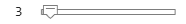3; [adsEnroll,adsTest,adsDET] = splitEachLabel(adsEvaluate,numFilesPerSpeakerForEnrollment,2);```

Display the label distributions of the resulting `audioDatastore` objects.

`countEachLabel(adsTrain)`
```ans=16×2 table Label Count _____ _____ F01 236 F02 236 F03 236 F04 236 F06 236 F07 236 F08 234 F09 236 M01 236 M02 236 M03 236 M04 236 M06 236 M07 236 M08 236 M09 236 ```
`countEachLabel(adsEnroll)`
```ans=2×2 table Label Count _____ _____ F05 3 M05 3 ```
`countEachLabel(adsDET)`
```ans=2×2 table Label Count _____ _____ F05 231 M05 231 ```
`countEachLabel(adsTest)`
```ans=2×2 table Label Count _____ _____ F05 2 M05 2 ```

Read an audio file from the training data set, listen to it, and plot it. Reset the datastore.

```[audio,audioInfo] = read(adsTrain); fs = audioInfo.SampleRate; t = (0:size(audio,1)-1)/fs; sound(audio,fs) plot(t,audio) xlabel('Time (s)') ylabel('Amplitude') axis([0 t(end) -1 1]) title('Sample Utterance from Training Set')````reset(adsTrain)`

You can reduce the data set and the number of parameters used in this example to speed up the runtime at the cost of performance. In general, reducing the data set is a good practice for development and debugging.

```speedUpExample =false; if speedUpExample adsTrain = splitEachLabel(adsTrain,30); adsDET = splitEachLabel(adsDET,21); end```

### Feature Extraction

Create an `audioFeatureExtractor` object to extract 20 MFCCs, 20 delta-MFCCs, and 20 delta-delta MFCCs. Use a delta window length of 9. Extract features from 25 ms Hann windows with a 10 ms hop.

```numCoeffs =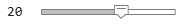20; deltaWindowLength =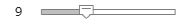9; windowDuration =0.025; hopDuration =0.01; windowSamples = round(windowDuration*fs); hopSamples = round(hopDuration*fs); overlapSamples = windowSamples - hopSamples; afe = audioFeatureExtractor( ... 'SampleRate',fs, ... 'Window',hann(windowSamples,'periodic'), ... 'OverlapLength',overlapSamples, ... ... 'mfcc',true, ... 'mfccDelta',true, ... 'mfccDeltaDelta',true); setExtractorParams(afe,'mfcc','DeltaWindowLength',deltaWindowLength,'NumCoeffs',numCoeffs)```

Extract features from the audio read from the training datastore. Features are returned as a `numHops`-by-`numFeatures` matrix.

```features = extract(afe,audio); [numHops,numFeatures] = size(features)```
```numHops = 797 ```
```numFeatures = 60 ```

### Training

Training an i-vector system is computationally expensive and time-consuming. If you have Parallel Computing Toolbox™, you can spread the work across multiple cores to speed up the example. Determine the optimal number of partitions for your system. If you do not have Parallel Computing Toolbox™, use a single partition.

```if ~isempty(ver('parallel')) && ~speedUpExample pool = gcp; numPar = numpartitions(adsTrain,pool); else numPar = 1; end```
```Starting parallel pool (parpool) using the 'local' profile ... Connected to the parallel pool (number of workers: 6). ```

### Feature Normalization Factors

Use the helper function, `helperFeatureExtraction`, to extract all features from the data set. The helperFeatureExtraction function extracts MFCC from regions of speech in the audio. The speech detection is performed by the `detectSpeech` function.

```featuresAll = {}; tic parfor ii = 1:numPar adsPart = partition(adsTrain,numPar,ii); featuresPart = cell(0,numel(adsPart.Files)); for iii = 1:numel(adsPart.Files) audioData = read(adsPart); featuresPart{iii} = helperFeatureExtraction(audioData,afe,[]); end featuresAll = [featuresAll,featuresPart]; end allFeatures = cat(2,featuresAll{:}); fprintf('Feature extraction from training set complete (%0.0f seconds).',toc)```
```Feature extraction from training set complete (58 seconds). ```

Calculate the global mean and standard deviation of each feature. You will use these in future calls to the `helperFeatureExtraction` function to normalize the features.

```normFactors.Mean = mean(allFeatures,2,'omitnan'); normFactors.STD = std(allFeatures,[],2,'omitnan');```

### Universal Background Model (UBM)

Initialize the Gaussian mixture model (GMM) that will be the universal background model (UBM) in the i-vector system. The component weights are initialized as evenly distributed. Systems trained on the TIMIT data set usually contain around 2048 components.

```numComponents =64; if speedUpExample numComponents = 32; end alpha = ones(1,numComponents)/numComponents; mu = randn(numFeatures,numComponents); vari = rand(numFeatures,numComponents) + eps; ubm = struct('ComponentProportion',alpha,'mu',mu,'sigma',vari);```

Train the UBM using the expectation-maximization (EM) algorithm.

```maxIter =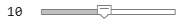10; if speedUpExample maxIter = 2; end tic for iter = 1:maxIter tic % EXPECTATION N = zeros(1,numComponents); F = zeros(numFeatures,numComponents); S = zeros(numFeatures,numComponents); L = 0; parfor ii = 1:numPar adsPart = partition(adsTrain,numPar,ii); while hasdata(adsPart) audioData = read(adsPart); % Extract features Y = helperFeatureExtraction(audioData,afe,normFactors); % Compute a posteriori log-liklihood logLikelihood = helperGMMLogLikelihood(Y,ubm); % Compute a posteriori normalized probability amax = max(logLikelihood,[],1); logLikelihoodSum = amax + log(sum(exp(logLikelihood-amax),1)); gamma = exp(logLikelihood - logLikelihoodSum)'; % Compute Baum-Welch statistics n = sum(gamma,1); f = Y * gamma; s = (Y.*Y) * gamma; % Update the sufficient statistics over utterances N = N + n; F = F + f; S = S + s; % Update the log-likelihood L = L + sum(logLikelihoodSum); end end % Print current log-likelihood fprintf('Training UBM: %d/%d complete (%0.0f seconds), Log-likelihood = %0.0f\n',iter,maxIter,toc,L) % MAXIMIZATION N = max(N,eps); ubm.ComponentProportion = max(N/sum(N),eps); ubm.ComponentProportion = ubm.ComponentProportion/sum(ubm.ComponentProportion); ubm.mu = F./N; ubm.sigma = max(S./N - ubm.mu.^2,eps); end```
```Training UBM: 1/10 complete (59 seconds), Log-likelihood = -162907120 Training UBM: 2/10 complete (53 seconds), Log-likelihood = -82282814 Training UBM: 3/10 complete (54 seconds), Log-likelihood = -78667384 Training UBM: 4/10 complete (55 seconds), Log-likelihood = -77041863 Training UBM: 5/10 complete (54 seconds), Log-likelihood = -76338342 Training UBM: 6/10 complete (52 seconds), Log-likelihood = -75958218 Training UBM: 7/10 complete (52 seconds), Log-likelihood = -75724712 Training UBM: 8/10 complete (53 seconds), Log-likelihood = -75561701 Training UBM: 9/10 complete (54 seconds), Log-likelihood = -75417170 Training UBM: 10/10 complete (55 seconds), Log-likelihood = -75275185 ```

### Calculate Baum-Welch Statistics

The Baum-Welch statistics are the `N` (zeroth order) and `F` (first order) statistics used in the EM algorithm, calculated using the final UBM.

`${\mathit{N}}_{\mathit{c}}\left(\mathit{s}\right)=\sum _{\mathit{t}}{\gamma }_{\mathit{t}}\left(\mathit{c}\right)$`

`${\mathit{F}}_{\mathit{c}}\left(\mathit{s}\right)=\sum _{\mathit{t}}{\gamma }_{\mathit{t}}\left(\mathit{c}\right){\mathit{Y}}_{\mathit{t}}$`

• ${\mathit{Y}}_{\mathit{t}}$ is the feature vector at time $\mathit{t}$.

• $\mathit{s}\in \left\{{\mathit{s}}_{1},{\mathit{s}}_{2,}...,{\mathit{s}}_{\mathit{N}}\right\}$, where $\mathit{N}$ is the number of speakers. For the purposes of training the total variability space, each audio file is considered a separate speaker (whether or not it belongs to a physical single speaker).

• ${\gamma }_{\mathit{t}}\left(\mathit{c}\right)$ is the posterior probability that the UBM component $\mathit{c}$ accounts for the feature vector ${\mathit{Y}}_{\mathit{t}}$.

Calculate the zeroth and first order Baum-Welch statistics over the training set.

```numSpeakers = numel(adsTrain.Files); Nc = {}; Fc = {}; tic parfor ii = 1:numPar adsPart = partition(adsTrain,numPar,ii); numFiles = numel(adsPart.Files); Npart = cell(1,numFiles); Fpart = cell(1,numFiles); for jj = 1:numFiles audioData = read(adsPart); % Extract features Y = helperFeatureExtraction(audioData,afe,normFactors); % Compute a posteriori log-likelihood logLikelihood = helperGMMLogLikelihood(Y,ubm); % Compute a posteriori normalized probability amax = max(logLikelihood,[],1); logLikelihoodSum = amax + log(sum(exp(logLikelihood-amax),1)); gamma = exp(logLikelihood - logLikelihoodSum)'; % Compute Baum-Welch statistics n = sum(gamma,1); f = Y * gamma; Npart{jj} = reshape(n,1,1,numComponents); Fpart{jj} = reshape(f,numFeatures,1,numComponents); end Nc = [Nc,Npart]; Fc = [Fc,Fpart]; end fprintf('Baum-Welch statistics completed (%0.0f seconds).\n',toc)```
```Baum-Welch statistics completed (54 seconds). ```

Expand the statistics into matrices and center $\mathit{F}\left(\mathit{s}\right)$, as described in , such that

• $\mathit{N}\left(\mathit{s}\right)$ is a $\mathit{C}\text{\hspace{0.17em}}\mathit{F}×\mathit{C}\text{\hspace{0.17em}}\mathit{F}$ diagonal matrix whose blocks are ${\mathit{N}}_{\mathit{c}}\left(\mathit{s}\right)\mathit{I}\text{\hspace{0.17em}}\left(\mathit{c}=1,...\mathit{C}\right)$.

• $\mathit{F}\left(\mathit{s}\right)$ is a $\mathit{C}\text{\hspace{0.17em}}\mathit{F}×1$ supervector obtained by concatenating ${\mathit{F}}_{\mathit{c}}\left(\mathit{s}\right)\text{\hspace{0.17em}\hspace{0.17em}}\left(\mathit{c}=1,...\mathit{C}\right)$.

• $\mathit{C}$ is the number of components in the UBM.

• $\mathit{F}$ is the number of features in a feature vector.

```N = Nc; F = Fc; muc = reshape(ubm.mu,numFeatures,1,[]); for s = 1:numSpeakers N{s} = repelem(reshape(Nc{s},1,[]),numFeatures); F{s} = reshape(Fc{s} - Nc{s}.*muc,[],1); end```

Because this example assumes a diagonal covariance matrix for the UBM, `N` are also diagonal matrices, and are saved as vectors for efficient computation.

### Total Variability Space

In the i-vector model, the ideal speaker supervector consists of a speaker-independent component and a speaker-dependent component. The speaker-dependent component consists of the total variability space model and the speaker's i-vector.

`$\mathit{M}=\mathit{m}+\mathit{Tw}$`

• $\mathit{M}$ is the speaker utterance supervector

• $\mathit{m}$ is the speaker- and channel-independent supervector, which can be taken to be the UBM supervector.

• $\mathit{T}$ is a low-rank rectangular matrix and represents the total variability subspace.

• $\mathit{w}$ is the i-vector for the speaker

The dimensionality of the i-vector, $\mathit{w}$, is typically much lower than the C F -dimensional speaker utterance supervector, making the i-vector, or i-vectors, a much more compact and tractable representation.

To train the total variability space, $\mathit{T}$, first randomly initialize `T`, then perform these steps iteratively :

1. Calculate the posterior distribution of the hidden variable.

`${\mathit{l}}_{\mathit{T}}\left(\mathit{s}\right)=\mathit{I}+{\mathit{T}}^{\prime }×{\Sigma }^{-1}×\mathit{N}\left(\mathit{s}\right)×\mathit{T}$`

2. Accumulate statistics across the speakers.

`$Κ=\sum _{\mathit{s}}\mathit{F}\left(\mathit{s}\right)×{\left({\mathit{l}}_{\mathit{T}}^{-1}\left(\mathit{s}\right)×{\mathit{T}}^{\prime }×{\Sigma }^{-1}×\mathit{F}\left(\mathit{s}\right)\right)}^{\prime }$`

`${\mathit{A}}_{\mathit{c}}=\sum _{\mathit{s}}{\mathit{N}}_{\mathit{c}}\left(\mathit{s}\right){\mathit{l}}_{\mathit{T}}^{-1}\left(\mathit{s}\right)$`

3. Update the total variability space.

`${\mathit{T}}_{\mathit{c}}={\mathit{A}}_{\mathit{c}}^{-1}×Κ$`

`$\mathit{T}=\left[\begin{array}{c}{\mathit{T}}_{1}\\ {\mathit{T}}_{2}\\ ⋮\\ {\mathit{T}}_{\mathit{C}}\end{array}\right]$`

 proposes initializing $\Sigma$ by the UBM variance, and then updating $\Sigma$ according to the equation:

`$\Sigma ={\left(\sum _{\mathit{s}}\mathit{N}\left(\mathit{s}\right)\right)}^{-1}\left(\left(\sum _{\mathit{s}}\mathit{S}\left(\mathit{s}\right)\right)-\mathrm{diag}\left(Κ×{\mathit{T}}^{\prime }\right)\right)$`

where S(s) is the centered second-order Baum-Welch statistic. However, updating $\Sigma$ is often dropped in practice as it has little effect. This example does not update $\Sigma$.

Create the sigma variable.

`Sigma = ubm.sigma(:);`

Specify the dimension of the total variability space. A typical value used for the TIMIT data set is 1000.

```numTdim =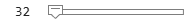32; if speedUpExample numTdim = 16; end```

Initialize `T` and the identity matrix, and preallocate cell arrays.

```T = randn(numel(ubm.sigma),numTdim); T = T/norm(T); I = eye(numTdim); Ey = cell(numSpeakers,1); Eyy = cell(numSpeakers,1); Linv = cell(numSpeakers,1);```

Set the number of iterations for training. A typical value reported is 20.

`numIterations =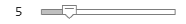5;`

Run the training loop.

```for iterIdx = 1:numIterations tic % 1. Calculate the posterior distribution of the hidden variable TtimesInverseSSdiag = (T./Sigma)'; parfor s = 1:numSpeakers L = (I + TtimesInverseSSdiag.*N{s}*T); Linv{s} = pinv(L); Ey{s} = Linv{s}*TtimesInverseSSdiag*F{s}; Eyy{s} = Linv{s} + Ey{s}*Ey{s}'; end % 2. Accumlate statistics across the speakers Eymat = cat(2,Ey{:}); FFmat = cat(2,F{:}); Kt = FFmat*Eymat'; K = mat2cell(Kt',numTdim,repelem(numFeatures,numComponents)); newT = cell(numComponents,1); for c = 1:numComponents AcLocal = zeros(numTdim); for s = 1:numSpeakers AcLocal = AcLocal + Nc{s}(:,:,c)*Eyy{s}; end % 3. Update the Total Variability Space newT{c} = (pinv(AcLocal)*K{c})'; end T = cat(1,newT{:}); fprintf('Training Total Variability Space: %d/%d complete (%0.0f seconds).\n',iterIdx,numIterations,toc) end```
```Training Total Variability Space: 1/5 complete (2 seconds). Training Total Variability Space: 2/5 complete (2 seconds). Training Total Variability Space: 3/5 complete (2 seconds). Training Total Variability Space: 4/5 complete (1 seconds). Training Total Variability Space: 5/5 complete (1 seconds). ```

### i-Vector Extraction

Once the total variability space is calculated, you can calculate the i-vectors as :

`$\mathit{w}={\left(\mathit{I}+{\mathit{T}}^{\prime }{\Sigma }^{-1\text{\hspace{0.17em}}}\mathrm{NT}\right)}^{\prime }{\mathit{T}}^{\prime }{\Sigma }^{-1\text{\hspace{0.17em}}}\mathit{F}$`

At this point, you are still considering each training file as a separate speaker. However, in the next step, when you train a projection matrix to reduce dimensionality and increase inter-speaker differences, the i-vectors must be labeled with the appropriate, distinct speaker IDs.

Create a cell array where each element of the cell array contains a matrix of i-vectors across files for a particular speaker.

```speakers = unique(adsTrain.Labels); numSpeakers = numel(speakers); ivectorPerSpeaker = cell(numSpeakers,1); TS = T./Sigma; TSi = TS'; ubmMu = ubm.mu; tic parfor speakerIdx = 1:numSpeakers % Subset the datastore to the speaker you are adapting. adsPart = subset(adsTrain,adsTrain.Labels==speakers(speakerIdx)); numFiles = numel(adsPart.Files); ivectorPerFile = zeros(numTdim,numFiles); for fileIdx = 1:numFiles audioData = read(adsPart); % Extract features Y = helperFeatureExtraction(audioData,afe,normFactors); % Compute a posteriori log-likelihood logLikelihood = helperGMMLogLikelihood(Y,ubm); % Compute a posteriori normalized probability amax = max(logLikelihood,[],1); logLikelihoodSum = amax + log(sum(exp(logLikelihood-amax),1)); gamma = exp(logLikelihood - logLikelihoodSum)'; % Compute Baum-Welch statistics n = sum(gamma,1); f = Y * gamma - n.*(ubmMu); ivectorPerFile(:,fileIdx) = pinv(I + (TS.*repelem(n(:),numFeatures))' * T) * TSi * f(:); end ivectorPerSpeaker{speakerIdx} = ivectorPerFile; end fprintf('I-vectors extracted from training set (%0.0f seconds).\n',toc)```
```I-vectors extracted from training set (60 seconds). ```

### Projection Matrix

Many different backends have been proposed for i-vectors. The most straightforward and still well-performing one is the combination of linear discriminant analysis (LDA) and within-class covariance normalization (WCCN).

Create a matrix of the training vectors and a map indicating which i-vector corresponds to which speaker. Initialize the projection matrix as an identity matrix.

```w = ivectorPerSpeaker; utterancePerSpeaker = cellfun(@(x)size(x,2),w); ivectorsTrain = cat(2,w{:}); projectionMatrix = eye(size(w{1},1));```

LDA attempts to minimize the intra-class variance and maximize the variance between speakers. It can be calculated as outlined in :

Given:

`${\mathit{S}}_{\mathit{b}}=\sum _{\mathit{s}=1}^{\mathit{S}}\left(\stackrel{‾}{{\mathit{w}}_{\mathit{s}}}-\stackrel{‾}{\mathit{w}}\right){\left(\stackrel{‾}{{\mathit{w}}_{\mathit{s}}}-\stackrel{‾}{\mathit{w}}\right)}^{\prime }$`

`${\mathit{S}}_{\mathit{w}}=\sum _{\mathit{s}=1}^{\mathit{S}}\frac{1}{{\mathit{n}}_{\mathit{s}}}\sum _{\mathit{i}=1}^{{\mathit{n}}_{\mathit{s}}}\left({\mathit{w}}_{\mathit{i}}^{\mathit{s}}-\stackrel{‾}{{\mathit{w}}_{\mathit{s}}}\right){\left({\mathit{w}}_{\mathit{i}}^{\mathit{s}}-\stackrel{‾}{{\mathit{w}}_{\mathit{s}}}\right)}^{\prime }$`

where

• $\stackrel{‾}{{\mathit{w}}_{\mathit{s}}}=\left(\frac{1}{{\mathit{n}}_{\mathit{s}}}\right){\sum }_{\mathit{i}=1}^{{\mathit{n}}_{\mathit{s}}}{\mathit{w}}_{\mathit{i}}^{\mathit{s}}$ is the mean of i-vectors for each speaker.

• $\stackrel{‾}{\mathit{w}}=\frac{1}{\mathit{N}}{\sum }_{\mathit{s}=1}^{\mathit{S}}{\sum }_{\mathit{i}=1}^{{\mathit{n}}_{\mathit{s}}}{\mathit{w}}_{\mathit{i}}^{\mathit{s}}$ is the mean i-vector across all speakers.

• ${\mathit{n}}_{\mathit{s}}$ is the number of utterances for each speaker.

Solve the eigenvalue equation for the best eigenvectors:

`${\mathit{S}}_{\mathit{b}}\mathit{v}=\lambda \text{\hspace{0.17em}}{\mathit{S}}_{\mathit{w}}\mathit{v}$`

The best eigenvectors are those with the highest eigenvalues.

```performLDA =true; if performLDA tic numEigenvectors =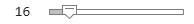16; Sw = zeros(size(projectionMatrix,1)); Sb = zeros(size(projectionMatrix,1)); wbar = mean(cat(2,w{:}),2); for ii = 1:numel(w) ws = w{ii}; wsbar = mean(ws,2); Sb = Sb + (wsbar - wbar)*(wsbar - wbar)'; Sw = Sw + cov(ws',1); end [A,~] = eigs(Sb,Sw,numEigenvectors); A = (A./vecnorm(A))'; ivectorsTrain = A * ivectorsTrain; w = mat2cell(ivectorsTrain,size(ivectorsTrain,1),utterancePerSpeaker); projectionMatrix = A * projectionMatrix; fprintf('LDA projection matrix calculated (%0.2f seconds).',toc) end```
```LDA projection matrix calculated (0.22 seconds). ```

WCCN attempts to scale the i-vector space inversely to the in-class covariance, so that directions of high intra-speaker variability are de-emphasized in i-vector comparisons .

Given the within-class covariance matrix:

`$\mathit{W}=\frac{1}{\mathit{S}}\sum _{\mathit{s}=1}^{\mathit{S}}\frac{1}{{\mathit{n}}_{\mathit{s}}}\sum _{\mathit{i}=1}^{{\mathit{n}}_{\mathit{s}}}\left({\mathit{w}}_{\mathit{i}}^{\mathit{s}}-\stackrel{‾}{{\mathit{w}}_{\mathit{s}}}\right){\left({\mathit{w}}_{\mathit{i}}^{\mathit{s}}-\stackrel{‾}{{\mathit{w}}_{\mathit{s}}}\right)}^{\prime }$`

where

• $\stackrel{‾}{{\mathit{w}}_{\mathit{s}}}=\left(\frac{1}{{\mathit{n}}_{\mathit{s}}}\right){\sum }_{\mathit{i}=1}^{{\mathit{n}}_{\mathit{s}}}{\mathit{w}}_{\mathit{i}}^{\mathit{s}}$ is the mean of i-vectors for each speaker.

• ${\mathit{n}}_{\mathit{s}}$ is the number of utterances for each speaker.

Solve for B using Cholesky decomposition:

`${\mathit{W}}^{-1}={\mathrm{BB}}^{\prime }$`

```performWCCN =true; if performWCCN tic alpha =0.9; W = zeros(size(projectionMatrix,1)); for ii = 1:numel(w) W = W + cov(w{ii}',1); end W = W/numel(w); W = (1 - alpha)*W + alpha*eye(size(W,1)); B = chol(pinv(W),'lower'); projectionMatrix = B * projectionMatrix; fprintf('WCCN projection matrix calculated (%0.4f seconds).',toc) end```
```WCCN projection matrix calculated (0.0063 seconds). ```

The training stage is now complete. You can now use the universal background model (UBM), total variability space (T), and projection matrix to enroll and verify speakers.

### Train G-PLDA Model

Apply the projection matrix to the train set.

`ivectors = cellfun(@(x)projectionMatrix*x,ivectorPerSpeaker,'UniformOutput',false);`

This algorithm implemented in this example is a Gaussian PLDA as outlined in . In the Gaussian PLDA, the i-vector is represented with the following equation:

`${\varphi }_{\mathrm{ij}}=\mu +{\mathrm{Vy}}_{\mathit{i}}+{\epsilon }_{\mathrm{ij}}$`

`${\mathit{y}}_{\mathit{i}}\sim Ν\left(0,Ι\right)$`

`${\epsilon }_{\mathrm{ij}}\sim Ν\left(0,{\Lambda }^{-1}\right)$`

where $\mu \text{\hspace{0.17em}}$is a global mean of the i-vectors, $\Lambda$ is a full precision matrix of the noise term ${\epsilon }_{\mathrm{ij}}$, and $\mathit{V}$ is the factor loading matrix, also known as the eigenvoices.

Specify the number of eigenvoices to use. Typically numbers are between 10 and 400.

`numEigenVoices =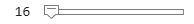16;`

Determine the number of disjoint persons, the number of dimensions in the feature vectors, and the number of utterances per speaker.

```K = numel(ivectors); D = size(ivectors{1},1); utterancePerSpeaker = cellfun(@(x)size(x,2),ivectors);```

Find the total number of samples and center the i-vectors.

`$\mathit{N}=\sum _{\mathit{i}=1}^{\mathit{K}}{\mathit{n}}_{\mathit{i}}$`

`$\mu =\frac{1}{\mathit{N}}\sum _{\mathit{i},\mathit{j}}{\varphi }_{\mathit{i},\mathit{j}}$`

`${\phi }_{\mathrm{ij}}={\varphi }_{\mathrm{ij}}-\mu$`

```ivectorsMatrix = cat(2,ivectors{:}); N = size(ivectorsMatrix,2); mu = mean(ivectorsMatrix,2); ivectorsMatrix = ivectorsMatrix - mu;```

Determine a whitening matrix from the training i-vectors and then whiten the i-vectors. Specify either ZCA whitening, PCA whitening, or no whitening.

```whiteningType =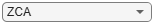'ZCA'; if strcmpi(whiteningType,'ZCA') S = cov(ivectorsMatrix'); [~,sD,sV] = svd(S); W = diag(1./(sqrt(diag(sD)) + eps))*sV'; ivectorsMatrix = W * ivectorsMatrix; elseif strcmpi(whiteningType,'PCA') S = cov(ivectorsMatrix'); [sV,sD] = eig(S); W = diag(1./(sqrt(diag(sD)) + eps))*sV'; ivectorsMatrix = W * ivectorsMatrix; else W = eye(size(ivectorsMatrix,1)); end```

Apply length normalization and then convert the training i-vector matrix back to a cell array.

`ivectorsMatrix = ivectorsMatrix./vecnorm(ivectorsMatrix);`

Compute the global second-order moment as

`$\mathit{S}=\sum _{\mathrm{ij}}{\phi }_{\mathrm{ij}}{\phi }_{\mathrm{ij}}^{\mathit{T}}$`

`S = ivectorsMatrix*ivectorsMatrix';`

Convert the training i-vector matrix back to a cell array.

`ivectors = mat2cell(ivectorsMatrix,D,utterancePerSpeaker);`

Sort persons according to the number of samples and then group the i-vectors by number of utterances per speaker. Precalculate the first-order moment of the $\mathit{i}$-th person as

`${\mathit{f}}_{\mathit{i}}=\sum _{\mathit{j}=1}^{{\mathit{n}}_{\mathit{i}}}{\phi }_{\mathrm{ij}}$`

```uniqueLengths = unique(utterancePerSpeaker); numUniqueLengths = numel(uniqueLengths); speakerIdx = 1; f = zeros(D,K); for uniqueLengthIdx = 1:numUniqueLengths idx = find(utterancePerSpeaker==uniqueLengths(uniqueLengthIdx)); temp = {}; for speakerIdxWithinUniqueLength = 1:numel(idx) rho = ivectors(idx(speakerIdxWithinUniqueLength)); temp = [temp;rho]; %#ok<AGROW> f(:,speakerIdx) = sum(rho{:},2); speakerIdx = speakerIdx+1; end ivectorsSorted{uniqueLengthIdx} = temp; %#ok<SAGROW> end```

Initialize the eigenvoices matrix, V, and the inverse noise variance term, $\Lambda$.

```V = randn(D,numEigenVoices); Lambda = pinv(S/N);```

Specify the number of iterations for the EM algorithm and whether or not to apply the minimum divergence.

```numIter =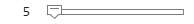5; minimumDivergence =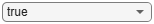true;```

Train the G-PLDA model using the EM algorithm described in .

```for iter = 1:numIter % EXPECTATION gamma = zeros(numEigenVoices,numEigenVoices); EyTotal = zeros(numEigenVoices,K); R = zeros(numEigenVoices,numEigenVoices); idx = 1; for lengthIndex = 1:numUniqueLengths ivectorLength = uniqueLengths(lengthIndex); % Isolate i-vectors of the same given length iv = ivectorsSorted{lengthIndex}; % Calculate M M = pinv(ivectorLength*(V'*(Lambda*V)) + eye(numEigenVoices)); % Equation (A.7) in  % Loop over each speaker for the current i-vector length for speakerIndex = 1:numel(iv) % First moment of latent variable for V Ey = M*V'*Lambda*f(:,idx); % Equation (A.8) in  % Calculate second moment. Eyy = Ey * Ey'; % Update Ryy R = R + ivectorLength*(M + Eyy); % Equation (A.13) in  % Append EyTotal EyTotal(:,idx) = Ey; idx = idx + 1; % If using minimum divergence, update gamma. if minimumDivergence gamma = gamma + (M + Eyy); % Equation (A.18) in  end end end % Calculate T TT = EyTotal*f'; % Equation (A.12) in  % MAXIMIZATION V = TT'*pinv(R); % Equation (A.16) in  Lambda = pinv((S - V*TT)/N); % Equation (A.17) in  % MINIMUM DIVERGENCE if minimumDivergence gamma = gamma/K; % Equation (A.18) in  V = V*chol(gamma,'lower');% Equation (A.22) in  end end```

Once you've trained the G-PLDA model, you can use it to calculate a score based on the log-likelihood ratio as described in . Given two i-vectors that have been centered, whitened, and length-normalized, the score is calculated as:

`$\mathrm{score}\left({\mathit{w}}_{1},{\mathit{w}}_{\mathit{t}}\right)=\left[\begin{array}{cc}{\mathit{w}}_{1}^{\mathit{T}}& {\mathit{w}}_{\mathit{t}}^{\mathit{T}}\end{array}\right]\left[\begin{array}{cc}\Sigma +{\mathrm{VV}}^{\mathit{T}}& {\mathrm{VV}}^{\mathit{T}}\\ {\mathrm{VV}}^{\mathit{T}}& \Sigma +{\mathrm{VV}}^{\mathit{T}}\end{array}\right]\left[\begin{array}{cc}{\mathit{w}}_{1}& {\mathit{w}}_{\mathit{t}}\end{array}\right]-{\mathit{w}}_{1}^{\mathit{T}}{\left[\begin{array}{c}\Sigma +{\mathrm{VV}}^{\mathit{T}}\end{array}\right]}^{-1}{\mathit{w}}_{1}-{\mathit{w}}_{\mathit{t}}^{\mathit{T}}{\left[\begin{array}{c}\Sigma +{\mathrm{VV}}^{\mathit{T}}\end{array}\right]}^{-1}{\mathit{w}}_{\mathit{t}}+\mathit{C}$`

where ${\mathit{w}}_{1}$ and ${\mathit{w}}_{\mathit{t}}$ are the enrollment and test i-vectors, $\Sigma$ is the variance matrix of the noise term, $\mathrm{V}$ is the eigenvoice matrix. The $\mathit{C}$ term are factored-out contants and can be dropped in practice.

```speakerIdx =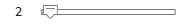2; utteranceIdx =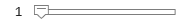1; w1 = ivectors{speakerIdx}(:,utteranceIdx); speakerIdx =1; utteranceIdx =10; wt = ivectors{speakerIdx}(:,utteranceIdx); VVt = V*V'; SigmaPlusVVt = pinv(Lambda) + VVt; term1 = pinv([SigmaPlusVVt VVt;VVt SigmaPlusVVt]); term2 = pinv(SigmaPlusVVt); w1wt = [w1;wt]; score = w1wt'*term1*w1wt - w1'*term2*w1 - wt'*term2*wt```
```score = 52.4507 ```

In pratice, the test i-vectors, and depending on your system, the enrollment ivectors, are not used in the training of the G-PLDA model. In the following evaluation section, you use previously unseen data for enrollment and verification. The supporting function, gpldaScore encapsulates the scoring steps above, and additionally performs centering, whitening, and normalization. Save the trained G-PLDA model as a struct for use with the supporting function `gpldaScore`.

```gpldaModel = struct('mu',mu, ... 'WhiteningMatrix',W, ... 'EigenVoices',V, ... 'Sigma',pinv(Lambda));```

### Enroll

Enroll new speakers that were not in the training data set.

Create i-vectors for each file for each speaker in the enroll set using the this sequence of steps:

1. Feature Extraction

2. Baum-Welch Statistics: Determine the zeroth and first order statistics

3. i-vector Extraction

4. Intersession compensation

Then average the i-vectors across files to create an i-vector model for the speaker. Repeat the for each speaker.

```speakers = unique(adsEnroll.Labels); numSpeakers = numel(speakers); enrolledSpeakersByIdx = cell(numSpeakers,1); tic parfor speakerIdx = 1:numSpeakers % Subset the datastore to the speaker you are adapting. adsPart = subset(adsEnroll,adsEnroll.Labels==speakers(speakerIdx)); numFiles = numel(adsPart.Files); ivectorMat = zeros(size(projectionMatrix,1),numFiles); for fileIdx = 1:numFiles audioData = read(adsPart); % Extract features Y = helperFeatureExtraction(audioData,afe,normFactors); % Compute a posteriori log-likelihood logLikelihood = helperGMMLogLikelihood(Y,ubm); % Compute a posteriori normalized probability amax = max(logLikelihood,[],1); logLikelihoodSum = amax + log(sum(exp(logLikelihood-amax),1)); gamma = exp(logLikelihood - logLikelihoodSum)'; % Compute Baum-Welch statistics n = sum(gamma,1); f = Y * gamma - n.*(ubmMu); %i-vector Extraction w = pinv(I + (TS.*repelem(n(:),numFeatures))' * T) * TSi * f(:); % Intersession Compensation w = projectionMatrix*w; ivectorMat(:,fileIdx) = w; end % i-vector model enrolledSpeakersByIdx{speakerIdx} = mean(ivectorMat,2); end fprintf('Speakers enrolled (%0.0f seconds).\n',toc)```
```Speakers enrolled (0 seconds). ```

For bookkeeping purposes, convert the cell array of i-vectors to a structure, with the speaker IDs as fields and the i-vectors as values

```enrolledSpeakers = struct; for s = 1:numSpeakers enrolledSpeakers.(string(speakers(s))) = enrolledSpeakersByIdx{s}; end```

### Verification

Specify either the CSS or G-PLDA scoring method.

`scoringMethod =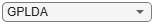'GPLDA';`

### False Rejection Rate (FRR)

The speaker false rejection rate (FRR) is the rate that a given speaker is incorrectly rejected. Create an array of scores for enrolled speaker i-vectors and i-vectors of the same speaker.

```speakersToTest = unique(adsDET.Labels); numSpeakers = numel(speakersToTest); scoreFRR = cell(numSpeakers,1); tic parfor speakerIdx = 1:numSpeakers adsPart = subset(adsDET,adsDET.Labels==speakersToTest(speakerIdx)); numFiles = numel(adsPart.Files); ivectorToTest = enrolledSpeakers.(string(speakersToTest(speakerIdx))); %#ok<PFBNS> score = zeros(numFiles,1); for fileIdx = 1:numFiles audioData = read(adsPart); % Extract features Y = helperFeatureExtraction(audioData,afe,normFactors); % Compute a posteriori log-likelihood logLikelihood = helperGMMLogLikelihood(Y,ubm); % Compute a posteriori normalized probability amax = max(logLikelihood,[],1); logLikelihoodSum = amax + log(sum(exp(logLikelihood-amax),1)); gamma = exp(logLikelihood - logLikelihoodSum)'; % Compute Baum-Welch statistics n = sum(gamma,1); f = Y * gamma - n.*(ubmMu); % Extract i-vector w = pinv(I + (TS.*repelem(n(:),numFeatures))' * T) * TSi * f(:); % Intersession Compensation w = projectionMatrix*w; % Score if strcmpi(scoringMethod,'CSS') score(fileIdx) = dot(ivectorToTest,w)/(norm(w)*norm(ivectorToTest)); else score(fileIdx) = gpldaScore(gpldaModel,w,ivectorToTest); end end scoreFRR{speakerIdx} = score; end fprintf('FRR calculated (%0.0f seconds).\n',toc)```
```FRR calculated (17 seconds). ```

### False Acceptance Rate (FAR)

The speaker false acceptance rate (FAR) is the rate that utterances not belonging to an enrolled speaker are incorrectly accepted as belonging to the enrolled speaker. Create an array of scores for enrolled speakers and i-vectors of different speakers.

```speakersToTest = unique(adsDET.Labels); numSpeakers = numel(speakersToTest); scoreFAR = cell(numSpeakers,1); tic parfor speakerIdx = 1:numSpeakers adsPart = subset(adsDET,adsDET.Labels~=speakersToTest(speakerIdx)); numFiles = numel(adsPart.Files); ivectorToTest = enrolledSpeakers.(string(speakersToTest(speakerIdx))); %#ok<PFBNS> score = zeros(numFiles,1); for fileIdx = 1:numFiles audioData = read(adsPart); % Extract features Y = helperFeatureExtraction(audioData,afe,normFactors); % Compute a posteriori log-likelihood logLikelihood = helperGMMLogLikelihood(Y,ubm); % Compute a posteriori normalized probability amax = max(logLikelihood,[],1); logLikelihoodSum = amax + log(sum(exp(logLikelihood-amax),1)); gamma = exp(logLikelihood - logLikelihoodSum)'; % Compute Baum-Welch statistics n = sum(gamma,1); f = Y * gamma - n.*(ubmMu); % Extract i-vector w = pinv(I + (TS.*repelem(n(:),numFeatures))' * T) * TSi * f(:); % Intersession compensation w = projectionMatrix * w; % Score if strcmpi(scoringMethod,'CSS') score(fileIdx) = dot(ivectorToTest,w)/(norm(w)*norm(ivectorToTest)); else score(fileIdx) = gpldaScore(gpldaModel,w,ivectorToTest); end end scoreFAR{speakerIdx} = score; end fprintf('FAR calculated (%0.0f seconds).\n',toc)```
```FAR calculated (17 seconds). ```

### Equal Error Rate (EER)

To compare multiple systems, you need a single metric that combines the FAR and FRR performance. For this, you determine the equal error rate (EER), which is the threshold where the FAR and FRR curves meet. In practice, the EER threshold might not be the best choice. For example, if speaker verification is used as part of a multi-authentication approach for wire transfers, FAR would most likely be more heavily weighted than FRR.

```amin = min(cat(1,scoreFRR{:},scoreFAR{:})); amax = max(cat(1,scoreFRR{:},scoreFAR{:})); thresholdsToTest = linspace(amin,amax,1000); % Compute the FRR and FAR for each of the thresholds. if strcmpi(scoringMethod,'CSS') % In CSS, a larger score indicates the enroll and test ivectors are % similar. FRR = mean(cat(1,scoreFRR{:})<thresholdsToTest); FAR = mean(cat(1,scoreFAR{:})>thresholdsToTest); else % In G-PLDA, a smaller score indicates the enroll and test ivectors are % similar. FRR = mean(cat(1,scoreFRR{:})>thresholdsToTest); FAR = mean(cat(1,scoreFAR{:})<thresholdsToTest); end [~,EERThresholdIdx] = min(abs(FAR - FRR)); EERThreshold = thresholdsToTest(EERThresholdIdx); EER = mean([FAR(EERThresholdIdx),FRR(EERThresholdIdx)]); figure plot(thresholdsToTest,FAR,'k', ... thresholdsToTest,FRR,'b', ... EERThreshold,EER,'ro','MarkerFaceColor','r') title(sprintf('Equal Error Rate = %0.4f, Threshold = %0.4f',EER,EERThreshold)) xlabel('Threshold') ylabel('Error Rate') legend('False Acceptance Rate (FAR)','False Rejection Rate (FRR)','Equal Error Rate (EER)','Location','best') grid on axis tight```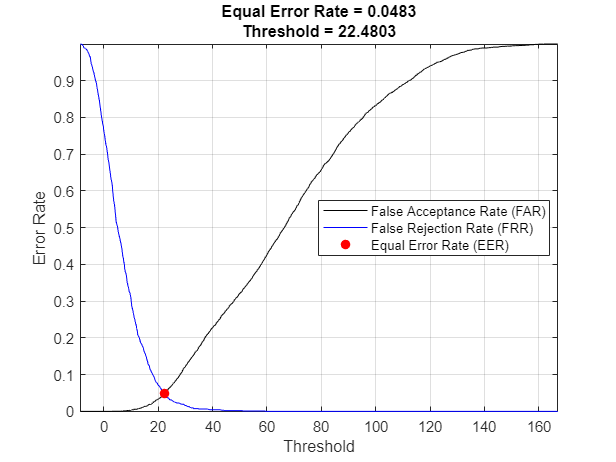### Feature Extraction and Normalization

```function [features,numFrames] = helperFeatureExtraction(audioData,afe,normFactors) % Input: % audioData - column vector of audio data % afe - audioFeatureExtractor object % normFactors - mean and standard deviation of the features used for normalization. % If normFactors is empty, no normalization is applied. % % Output % features - matrix of features extracted % numFrames - number of frames (feature vectors) returned % Normalize audioData = audioData/max(abs(audioData(:))); % Protect against NaNs audioData(isnan(audioData)) = 0; % Isolate speech segment idx = detectSpeech(audioData,afe.SampleRate); features = []; for ii = 1:size(idx,1) f = extract(afe,audioData(idx(ii,1):idx(ii,2))); features = [features;f]; %#ok<AGROW> end % Feature normalization if ~isempty(normFactors) features = (features-normFactors.Mean')./normFactors.STD'; end features = features'; % Cepstral mean subtraction (for channel noise) if ~isempty(normFactors) features = features - mean(features,'all'); end numFrames = size(features,2); end```

### Gaussian Multi-Component Mixture Log-Likelihood

```function L = helperGMMLogLikelihood(x,gmm) xMinusMu = repmat(x,1,1,numel(gmm.ComponentProportion)) - permute(gmm.mu,[1,3,2]); permuteSigma = permute(gmm.sigma,[1,3,2]); Lunweighted = -0.5*(sum(log(permuteSigma),1) + sum(xMinusMu.*(xMinusMu./permuteSigma),1) + size(gmm.mu,1)*log(2*pi)); temp = squeeze(permute(Lunweighted,[1,3,2])); if size(temp,1)==1 % If there is only one frame, the trailing singleton dimension was % removed in the permute. This accounts for that edge case. temp = temp'; end L = temp + log(gmm.ComponentProportion)'; end```

### G-PLDA Score

```function score = gpldaScore(gpldaModel,w1,wt) % Center the data w1 = w1 - gpldaModel.mu; wt = wt - gpldaModel.mu; % Whiten the data w1 = gpldaModel.WhiteningMatrix*w1; wt = gpldaModel.WhiteningMatrix*wt; % Length-normalize the data w1 = w1./vecnorm(w1); wt = wt./vecnorm(wt); % Score the similarity of the i-vectors based on the log-likelihood. VVt = gpldaModel.EigenVoices * gpldaModel.EigenVoices'; SVVt = gpldaModel.Sigma + VVt; term1 = pinv([SVVt VVt;VVt SVVt]); term2 = pinv(SVVt); w1wt = [w1;wt]; score = w1wt'*term1*w1wt - w1'*term2*w1 - wt'*term2*wt; end```

### References

 Reynolds, Douglas A., et al. “Speaker Verification Using Adapted Gaussian Mixture Models.” Digital Signal Processing, vol. 10, no. 1–3, Jan. 2000, pp. 19–41. DOI.org (Crossref), doi:10.1006/dspr.1999.0361.

 Kenny, Patrick, et al. “Joint Factor Analysis Versus Eigenchannels in Speaker Recognition.” IEEE Transactions on Audio, Speech and Language Processing, vol. 15, no. 4, May 2007, pp. 1435–47. DOI.org (Crossref), doi:10.1109/TASL.2006.881693.

 Kenny, P., et al. “A Study of Interspeaker Variability in Speaker Verification.” IEEE Transactions on Audio, Speech, and Language Processing, vol. 16, no. 5, July 2008, pp. 980–88. DOI.org (Crossref), doi:10.1109/TASL.2008.925147.

 Dehak, Najim, et al. “Front-End Factor Analysis for Speaker Verification.” IEEE Transactions on Audio, Speech, and Language Processing, vol. 19, no. 4, May 2011, pp. 788–98. DOI.org (Crossref), doi:10.1109/TASL.2010.2064307.

 Matejka, Pavel, Ondrej Glembek, Fabio Castaldo, M.j. Alam, Oldrich Plchot, Patrick Kenny, Lukas Burget, and Jan Cernocky. “Full-Covariance UBM and Heavy-Tailed PLDA in i-Vector Speaker Verification.” 2011 IEEE International Conference on Acoustics, Speech and Signal Processing (ICASSP), 2011. https://doi.org/10.1109/icassp.2011.5947436.

 Snyder, David, et al. “X-Vectors: Robust DNN Embeddings for Speaker Recognition.” 2018 IEEE International Conference on Acoustics, Speech and Signal Processing (ICASSP), IEEE, 2018, pp. 5329–33. DOI.org (Crossref), doi:10.1109/ICASSP.2018.8461375.

 Signal Processing and Speech Communication Laboratory. Accessed December 12, 2019. https://www.spsc.tugraz.at/databases-and-tools/ptdb-tug-pitch-tracking-database-from-graz-university-of-technology.html.

 Variani, Ehsan, et al. “Deep Neural Networks for Small Footprint Text-Dependent Speaker Verification.” 2014 IEEE International Conference on Acoustics, Speech and Signal Processing (ICASSP), IEEE, 2014, pp. 4052–56. DOI.org (Crossref), doi:10.1109/ICASSP.2014.6854363.

 Dehak, Najim, Réda Dehak, James R. Glass, Douglas A. Reynolds and Patrick Kenny. “Cosine Similarity Scoring without Score Normalization Techniques.” Odyssey (2010).

 Verma, Pulkit, and Pradip K. Das. “I-Vectors in Speech Processing Applications: A Survey.” International Journal of Speech Technology, vol. 18, no. 4, Dec. 2015, pp. 529–46. DOI.org (Crossref), doi:10.1007/s10772-015-9295-3.

 D. Garcia-Romero and C. Espy-Wilson, “Analysis of I-vector Length Normalization in Speaker Recognition Systems.” Interspeech, 2011, pp. 249–252.

 Kenny, Patrick. "Bayesian Speaker Verification with Heavy-Tailed Priors". Odyssey 2010 - The Speaker and Language Recognition Workshop, Brno, Czech Republic, 2010.

 Sizov, Aleksandr, Kong Aik Lee, and Tomi Kinnunen. “Unifying Probabilistic Linear Discriminant Analysis Variants in Biometric Authentication.” Lecture Notes in Computer Science Structural, Syntactic, and Statistical Pattern Recognition, 2014, 464–75. https://doi.org/10.1007/978-3-662-44415-3_47.

 Rajan, Padmanabhan, Anton Afanasyev, Ville Hautamäki, and Tomi Kinnunen. 2014. “From Single to Multiple Enrollment I-Vectors: Practical PLDA Scoring Variants for Speaker Verification.” Digital Signal Processing 31 (August): 93–101. https://doi.org/10.1016/j.dsp.2014.05.001.

## SupportGet trial now